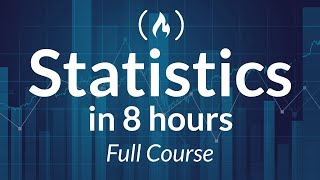# Statistics - A Full University Course on Data Science Basics

Learn the essentials of statistics in this complete course. This course introduces the various methods used to collect, organize, summarize, interpret and reach conclusions about data. An emphasis is placed on demonstrating that statistics is more than mathematical calculations. By using examples...
Learn the essentials of statistics in this complete course. This course introduces the various methods used to collect, organize, summarize, interpret and reach conclusions about data. An emphasis is placed on demonstrating that statistics is more than mathematical calculations. By using examples gathered from real life, students learn to use statistical methods as analytical tools to develop generalizations and meaningful conclusions in their field of study.

🎥 Course by Monika Wahi.
🔗 These lectures are based on the textbook Understanding Basic Statistics, 6th Edition, by Brase & Brase, available here: https://www.amazon.com/Understanding-Basic-Statistics-Charles-Henry/dp/1111827028
🔗 Monika Wahi's LinkedIn Learning courses are here: https://www.linkedin.com/learning/instructors/monika-wahi?u=2125562
🔗 Visit Monika Wahi's web page here: http://www.dethwench.com/
🔗 Monika Wahi's peer-reviewed articles listed here: https://scholar.google.com/citations?user=v3BDf1oAAAAJ&hl=en

⭐️ Course Contents ⭐️
⌨️ (0:00:00) What is statistics
⌨️ (0:33:34) Sampling
⌨️ (1:21:20) Experimental design
⌨️ (2:00:32) Randomization
⌨️ (2:16:25) Frequency histogram and distribution
⌨️ (2:35:58) Time series, bar and pie graphs
⌨️ (3:10:10) Frequency table and stem-and-leaf
⌨️ (3:39:41) Measures of central tendency
⌨️ (4:11:56) Measure of variation
⌨️ (4:58:35) Percentile and box-and-whisker plots
⌨️ (5:24:58) Scatter diagrams and linear correlation
⌨️ (6:39:54) Normal distribution and empirical rule
⌨️ (7:05:39) Z-score and probabilities
⌨️ (7:45:11) Sampling distributions and the central limit theorem

--

Learn to code for free and get a developer job: https://www.freecodecamp.org

Read hundreds of articles on programming: https://www.freecodecamp.org/news

#statistics #statistics full course #statistics tutorial #statistics for machine learning #statistics course #statistics tutorial for data science #statistics mean median mode #statistics for beginners #data science